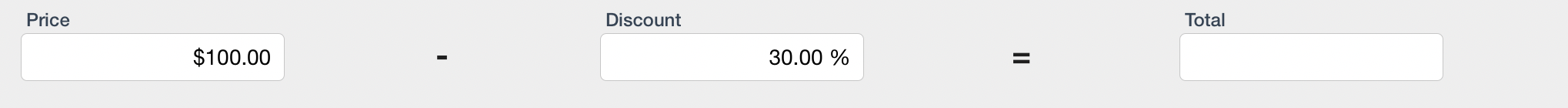0# Calculate Discount PercentageThis has been driving me crazy. I am trying to calculate the total after taking 30% OFF of the price to get the total, not 30% of the total.

I've tried Total := Price * Discount / 100 although that is only calculating the percentage of price, I am trying to calculate what the value would be with so much percentage off. Please help.

2replies Oldest first
• Oldest first